# 第二章. Javascript

• 修改页面内容

js 代码位置

``````<script>
// js 代码
</script>
``````

``````<script src="js脚本路径"></script>
``````
• 注意，到了框架之后，引入方式会有不同

## 1. 变量与数据类型

### 声明变量

#### 1) let ⭐

``````let 变量名 = 值;
``````
• let 声明的变量可以被多次赋值，例如
``````let a = 100;  // 初始值是 100
a = 200;	  // ok, 被重新赋值为 200
``````

#### 2) const ⭐

• const 修饰的叫常量，只能赋值一次
``````const b = 300; // 初始值是 300
b = 400;	   // error, 不能再次赋值
``````
• const 并不意味着它引用的内容不可修改，例如
``````const c = [1,2,3];
c = 4; 	        // ok, 数组内容被修改成 [1,2,4]
c = [5,6];			// error, 不能再次赋值
``````

#### 3) var

var 声明的变量可以被多次赋值，例如

``````var f = 100;
f = 200;
``````

### 基本类型

#### 1,2) undefined 和 null

• 执行表达式或函数，没有返回结果，出现 undefined
• 访问数组不存在的元素，访问对象不存在的属性，出现 undefined
• 定义变量，没有初始化，出现 undefined

``````console.log(1);  	// 函数没有返回值, 结果是  undefined
let a = 10;		 	// 表达式没有返回值, 结果是 undefined
let b = [1,2,3];
console.log(b); // 数组未定义元素是 undefined
let c = {"name":"张三"};
console.log(c.age); // 对象未定义属性是 undefined
let d;
console.log(d);		// 变量未初始化是 undefined
``````

• 都没有属性、方法
• 二者合称 Nullish

• undefined 由 js 产生
• null 由程序员提供

#### 3) string ⭐

js 字符串三种写法

``````let a = "hello";  // 双引号
let b = "world";  // 单引号
let c = `hello`;  // 反引号
``````

html 代码如下，用 java 和 js 中的字符串如何表示？

``````<a href="1.html">超链接</a>
``````

java 显得比较繁琐

``````String s1 = "<a href=\"1.html\">超链接</a>";

String s2 = """
<a href="1.html">超链接</a>""";
``````

js 就比较灵活

``````let s1 = '<a href="1.html">超链接</a>';

let s2 = `<a href="1.html">超链接</a>`;
``````

``````/test?name=zhang&age=18
/test?name=li&age=20
``````

``````let name = ; // zhang li ...
let age = ; // 18 20 ...

let uri = "/test?name=" + name + "&age=" + age;
``````

``````let name = ; // zhang li ...
let age = ; // 18 20 ...

let uri = `/test?name=\${name}&age=\${age}`;
``````

#### 4,5) number 和 bigint⭐

number 类型标识的是双精度浮动小数，例如

``````10 / 3;   // 结果 3.3333333333333335
``````

``````10 / 0;	  // 结果 Infinity 正无穷大
-10 / 0;  // 结果 -Infinity 负无穷大
``````

``````2.0 - 1.1; // 结果 0.8999999999999999
``````

``````parseInt("10"); 	// 结果是数字 10
parseInt("10.5");	// 结果是数字 10, 去除了小数部分
parseInt("10") / 3; // 结果仍视为 number 浮点数, 因此结果为 3.3333333333333335

parseInt("abc");	// 转换失败，结果是特殊值 NaN (Not a Number)
``````

``````10n / 3n;			// 结果 3n, 按整数除法处理
``````

#### 6) boolean ⭐

• Truthy
• Falsy

``````let b = 1;

if(b) { // true
console.log("进入了");
}
``````

• `false`
• `Nullish (null, undefined)`
• `0, 0n, NaN`
• `"" '' ``` 即长度为零的字符串

• `"false", "0"` 即字符串的 false 和 字符串的零
• `[]` 空数组
• `{}` 空对象

• 很少使用

### 对象类型

#### 1) Function ⭐⭐

##### 定义函数
``````function 函数名(参数) {
// 函数体
return 结果;
}
``````

``````function add(a, b) {
return a + b;
}
``````
##### 调用函数
``````函数名(实参);
``````

``````add(1, 2);     // 返回 3
``````

js 中的函数调用特点：对参数的类型个数都没有限制，例如

``````add('a', 'b');  // 返回 ab
add(4, 5, 6);   // 返回 9, 第三个参数没有被用到, 不会报错
add(1);			// 返回 NaN, 这时 b 没有定义是 undefined, undefined 做数学运算结果就是 NaN
``````
##### 默认参数

java 中（spring）要实现默认参数的效果得这么做：

``````@RestController
public class MyController {

@RequestMapping("/page")
@ResponseBody
public void page(
@RequestParam(defaultValue="1") int page,
@RequestParam(defaultValue="10") int size
){
// ...
}
}
``````

js

``````function pagination(page = 1, size = 10) {
console.log(page, size);
}
``````
##### 匿名函数

``````(function (参数) {
// 函数体
return 结果;
})
``````

``````(function(a,b){
return a + b;
})
``````

``````(function(a,b){
return a + b;
})(1,2)
``````

``````<p id="p1">点我啊</p>
``````

``````document.getElementById("p1").onclick = (function(){
console.log("鼠标单击了...");
});
``````
##### 箭头函数
``````(参数) => {
// 函数体
return 结果;
}
``````
• 如果没有参数，() 还是要保留
• 如果只有一个参数，() 可以省略
• 如果函数体内只有一行代码，{} 可以省略
• 如果这一行代码就是结果，return 可以省略

``````document.getElementById("p1").onclick = () =>  console.log("aa");
``````
##### 函数是对象

1. 可以参与赋值，例，具名函数也能参与赋值
``````function abc() {
console.log("bb");
}

document.getElementById("p1").onclick = abc;
``````
1. 有属性、有方法，执行 `console.dir(abc)`，输出结果如下
``````ƒ abc()
arguments: null
caller: null
length: 0
name: "abc"
➡prototype: {constructor: ƒ}
[[FunctionLocation]]: VM1962:1
➡[[Prototype]]: ƒ ()
➡[[Scopes]]: Scopes
``````
• 其中带有 f 标记的是方法，不带的是属性

• 带有 ➡ 符号的可以继续展开，限于篇幅省略了

• 带有 `[[ ]]` 的是内置属性，不能访问，只能查看

• 相对重要的是 `[[Prototype]]``[[Scopes]]` 会在后面继承和作用域时讲到

1. 可以作为方法参数
``````function a() {
console.log('a')
}

function b(fn) {          // fn 将来可以是一个函数对象
console.log('b')
fn();                 // 调用函数对象
}

b(a)
``````
1. 可以作为方法返回值
``````function c() {
console.log("c");
function d() {
console.log("d");
}
return d;
}

c()()
``````
##### 函数作用域

``````function a() {
function b() {
}
}
``````

``````function c() {
var z = 30;
}

var x = 10;
function a() {
var y = 20;
function b() {
// 看这里
console.log(x, y);
}
b();
}
a();
``````
• 以函数为分界线划定作用域，所有函数之外是全局作用域
• 查找变量时，由内向外查找
• 在内层作用域找到变量，就会停止查找，不会再找外层
• 所有作用域都找不到变量，报错
• 作用域本质上是函数对象的属性，可以通过 console.dir 来查看调试
##### 闭包
``````var x = 10;
function a() {
var y = 20;
function b() {
console.log(x,y);
}
return b;
}
a()();  // 在外面执行了 b
``````
• 函数定义时，它的作用域已经确定好了，因此无论函数将来去了哪，都能从它的作用域中找到当时那些变量
• 别被概念忽悠了，闭包就是指函数能够访问自己的作用域中变量
##### let、var 与作用域

``````let x = 10;
if(true) {
let y = 20;
function b() {
console.log(x,y);
}
console.dir(b);
}
``````

``````var x = 10;
if(true) {
var y = 20;
function b() {
console.log(x,y);
}
console.dir(b);
}
``````

``````var e = 10;
if(true) {
var e = 20;
console.log(e);	// 打印 20
}
console.log(e);		// 因为是同一个变量，还是打印 20
``````

``````let e = 10;
if(true) {
let e = 20;
console.log(e);	// 打印 20
}
console.log(e);		// 打印 10
``````

``````var e = 10;
if(true) {
function b() {
var e = 20;
console.log(e);
}
b();
}
console.log(e);
``````

#### 2) Array ⭐

``````// 创建数组
let arr = [1,2,3];

// 获取数组元素
console.log(arr); // 输出 1

// 修改数组元素
array = 5;		 // 数组元素变成了 [5,2,3]

// 遍历数组元素，其中 length 是数组属性，代表数组长度
for(let i = 0; i < arr.length; i++) {
console.log(arr[i]);
}
``````

API

• push、shift、splice
``````let arr = [1,2,3];

arr.push(4);    	// 向数组尾部(右侧)添加元素, 结果 [1,2,3,4]
arr.shift();		// 从数组头部(左侧)移除元素, 结果 [2,3,4]
arr.splice(1,1);	// 删除【参数1】索引位置的【参数2】个元素，结果 [2,4]
``````
• join
``````let arr = ['a','b','c'];

arr.join(); 		// 默认使用【,】作为连接符，结果 'a,b,c'
arr.join('');		// 结果 'abc'
arr.join('-');		// 结果 'a-b-c'
``````
• map、filter、forEach
``````let arr = [1,2,3,6];

function a(i) {   // 代表的新旧元素之间的变换规则
return i * 10
}

// arr.map(a) // 具名函数，结果 [10,20,30,60]

// arr.map( (i) => {return i * 10} ); // 箭头函数
arr.map( i => i * 10 ); // 箭头函数
``````
• 传给 map 的函数，参数代表旧元素，返回值代表新元素

map 的内部实现（伪代码）

``````function map(a) { // 参数是一个函数
let narr = [];
for(let i = 0; i < arr.length; i++) {
let o = arr[i]; // 旧元素
let n = a(o);   // 新元素
narr.push(n);
}
return narr;
}
``````

filter 例子

``````let arr = [1,2,3,6];
arr.filter( (i)=> i % 2 == 1 ); // 结果 [1,3]
``````
• 传给 filter 的函数，参数代表旧元素，返回 true 表示要留下的元素

forEach 例子

``````let arr = [1,2,3,6];

/*for(let i = 0; i < arr.length; i++) {
console.log(arr[i]);
}*/

arr.forEach( (i) => console.log(i) );
``````

• 高阶函数，map，filter，forEach
• 回调函数，例如作为参数传入的函数

#### 3) Object ⭐⭐

##### 语法
``````let obj = {
属性名: 值,
方法名: 函数,
get 属性名() {},
set 属性名(新值) {}
}
``````

``````let stu1 = {
name: "小明",
age: 18,
study: function(){
console.log(this.name + "爱学习");
}
}
``````

``````let name = "小黑";
let age = 20;
let study = function(){
console.log(this.name + "爱学习");
}

let stu2 = { name, age, study }
``````

``````let stu3 = {
name: "小白",
age: 18,
study(){
console.log(this.name + "爱学习");
}
}
``````
• 注意：对象方法这么写，仅限于对象内部

``````let stu4 = {
_name: null, /*类似于java中私有成员变量*/
get name() {
console.log("进入了get");
return this._name;
},
set name(name) {
console.log("进入了set");
this._name = name;
}
}
``````

``````stu4.name = "小白"

console.log(stu4.name)
``````
##### 特色：属性增删

• Java 的 Object 是以类作为模板来创建，对象不能脱离类模板的范围，一个对象的属性、能用的方法都是确定好的
• js 的对象，不需要什么模板，它的属性和方法可以随时加减
``````let stu = {name:'张三'};
stu.age = 18;					// 添加属性
delete stu.age;					// 删除属性

stu.study = function() {		// 添加方法
console.log(this.name + "在学习");
}
``````

``````let stu = {_name:null};

Object.defineProperty(stu, "name", {
get(){
return this._name;
},
set(name){
this._name = name;
}
});
``````
• 参数1：目标对象
• 参数2：属性名
• 参数3：get，set 的定义
##### 特色：this

``````public class TestMethod {

static class Student {
private String name;

public Student(String name) {
this.name = name;
}

public void study(Student this, String subject) {
System.out.println(this.name + "在学习 " + subject);
}
}

public static void main(String[] args) {
Student stu = new Student("小明");

// 下面的代码，本质上是执行 study(stu, "java")，因此 this 就是 stu
stu.study("java");
}
}
``````
• Java 中的 this 是个隐式参数
• Java 中，我们说 this 代表的就是调用方法的那个对象

js 中的 this 也是隐式参数，但它与函数运行时上下文相关

``````function study(subject) {
console.log(this.name + "在学习 " + subject)
}
``````

``````study("js");  // 输出 在学习 js
``````

``````let stu = {
name:"小白",
study
}
``````

``````stu.study('js'); 	// 输出 小白在学习 js
``````

``````let stu = {name:"小黑"};
study.call(stu, "js");	// 输出 小黑在学习 js
``````

``````let stu = {
name: "小花",
friends: ["小白","小黑","小明"],
play() {
this.friends.forEach(function(e){
console.log(this.name + "与" + e + "在玩耍");
});
}
}
stu.play()
``````
• this.name 所在的函数是【落单】的函数，因此 this 代表 window

``````与小白在玩耍

``````

``````let stu = {
name: "小花",
friends: ["小白","小黑","小明"],
play() {
this.friends.forEach(e => {
console.log(this.name + "与" + e + "在玩耍");
})
}
}
``````
• this.name 所在的函数是箭头函数，因此 this 要看它外层的 play 函数，play 又是属于 stu 的方法，因此 this 代表 stu 对象

``````小花与小白在玩耍

``````

``````let stu = {
name: "小花",
friends: ["小白","小黑","小明"],
play() {
let me = this;
this.friends.forEach(function(e){
console.log(me.name + "与" + e + "在玩耍");
});
}
}
``````
##### 特色：原型继承
``````let father = {
f1: '父属性',
m1: function() {
console.log("父方法");
}
}

let son = Object.create(father);

console.log(son.f1);  // 打印 父属性
son.m1();			  // 打印 父方法
``````
• father 是父对象，son 去调用 .m1 或 .f1 时，自身对象没有，就到父对象找
• son 自己可以添加自己的属性和方法
• son 里有特殊属性 `__proto__` 代表它的父对象，js 术语： son 的原型对象
• 不同浏览器对打印 son 的 `__proto__` 属性时显示不同
• Edge 打印 console.dir(son) 显示 `[[Prototype]]`
• Firefox 打印 console.dir(son) 显示 `<prototype>`
##### 特色：基于函数的原型继承

1. 负责创建子对象，给子对象提供属性、方法，功能上相当于构造方法

2. 函数有个特殊的属性 prototype，它就是函数创建的子对象的父对象

**注意！**名字有差异，这个属性的作用就是为新对象提供原型

``````function cons(f2) {
// 创建子对象(this), 给子对象提供属性和方法
this.f2 = f2;
this.m2 = function () {
console.log("子方法");
}
}
// cons.prototype 就是父对象
cons.prototype.f1 = "父属性";
cons.prototype.m1 = function() {
console.log("父方法");
}
``````

``````let son = new cons("子属性")
``````

##### JSON

``````{
"name":"张三",
"age":18
}
``````

``````{
name:"张三",
age:18
}
``````

1. 本质不同
• json 对象本质上是个字符串，它的职责是作为客户端和服务器之间传递数据的一种格式，它的属性只是样子货
• js 对象是切切实实的对象，可以有属性方法
2. 语法细节不同
• json 中只能有 null、true|false、数字、字符串（只有双引号）、对象、数组
• json 中不能有除以上的其它 js 对象的特性，如方法等
• json 中的属性必须用双引号引起来

json 字符串与 js 对象的转换

``````JSON.parse(json字符串);  // 返回js对象
JSON.stringify(js对象);  // 返回json字符串
``````

### 动态类型

``````int a = 10;
String b = "abc";

int c = "abc";  // 错误
``````

``````let a = 200;

let b = 100;
b = 'abc';
b = true;
``````

``````function test(obj) {
// obj 的类型未知，必须根据不同类型做出相应的容错处理
}
``````

## 2. 运算符与表达式

• `+ - * / % ** `
• `+= -= *= /= %= **=`
• `++ --`
• 位运算、移位运算
• `== != > >= < <=`
• `=== !==`
• `&& || !`
• `?? ?.`
• `...`
• 解构赋值 ⭐

### 1) ===

``````1 == 1    	// 返回 true
1 == '1'	// 返回 true，会先将右侧的字符串转为数字，再做比较
1 === '1'	// 返回 false，类型不等，直接返回 false
``````

typeof 查看某个值的类型

``````typeof 1	// 返回 'number'
typeof '1'	// 返回 'string'
``````

### 2) ||

``````function test(n = '男') {
console.log(n);
}
``````

``````function test(n) {
if(n === undefined) {
n = '男';
}
console.log(n);
}
``````

``````function test(n) {
n = (n === undefined) ? '男' : n;
console.log(n);
}
``````

``````function test(n) {
n = n || '男';
console.log(n);
}
``````

``````值1 || 值2
``````

### 3) ?? 与 ?.

#### ??

``````function test(n) {
if(n === undefined || n === null) {
n = '男';
}
console.log(n);
}
``````

``````function test(n) {
n = n ?? '男';
console.log(n);
}
``````

``````值1 ?? 值2
``````
• 值1 是 nullish，返回值2
• 值1 不是 nullish，返回值1

#### ?.

``````let stu1 = {
name:"张三",
city: '北京'
}
};

let stu2 = {
name:"李四"
}

let stu3 = {
name:"李四",
}
``````

``````function test(stu) {
}
``````

``````function test(stu) {
}
``````

``````function test(stu) {
console.log(undefined);
return;
}
}
``````

### 4) …

``````let arr = [1,2,3];

function test(a,b,c) {
console.log(a,b,c);
}
``````

``````test(arr,arr,arr);		// 输出 1,2,3
``````

``````test(...arr);					// 输出 1,2,3
``````
• 打散可以理解为【去掉了】数组外侧的中括号，只剩下数组元素

``````let arr1 = [1,2,3];
let arr2 = [...arr1];		// 复制数组
``````

``````let obj1 = {name:'张三', age: 18};

let obj2 = {...obj1};		// 复制对象
``````

``````let o1 = {name:'张三', address: {city: '北京'} }

let o2 = {...o1};
``````

``````let a1 = [1,2];
let a2 = [3,4];

let b1 = [...a1,...a2];		// 结果 [1,2,3,4]
let b2 = [...a2,5,...a1]	// 结果 [3,4,5,1,2]
``````

``````let o1 = {name:'张三'};
let o2 = {age:18};
let o3 = {name:'李四'};

let n1 = {...o1, ...o2};	// 结果 {name:'张三',age:18}

let n2 = {...o3, ...o2, ...o1}; // 结果{name:'李四',age:18}
``````
• 复制对象时出现同名属性，后面的会覆盖前面的

### 5) [] {}

#### []

``````let arr = [1,2,3];

let [a, b, c] = arr;	// 结果 a=1, b=2, c=3
``````

``````let arr = [1,2,3];

function test([a,b,c]) {
console.log(a,b,c) 	// 结果 a=1, b=2, c=3
}

test(arr);
``````

#### {}

``````let obj = {name:"张三", age:18};

let {name,age} = obj;	// 结果 name=张三, age=18
``````

``````let obj = {name:"张三", age:18};

function test({name, age}) {
console.log(name, age); // 结果 name=张三, age=18
}

test(obj)
``````

## 3. 控制语句

• `if ... else`
• `switch`
• `while`
• `do ... while`
• `for`
• `for ... in`
• `for ... of`
• `try ... catch`

### 1) for in

``````let father = {name:'张三', age:18, study:function(){}};

for(const n in father) {
console.log(n);
}
``````
• 其中 const n 代表遍历出来的属性名
• 注意1：方法名也能被遍历出来（它其实也算一种特殊属性）
• 注意2：遍历子对象时，父对象的属性会跟着遍历出来
``````let son = Object.create(father);
son.sex = "男";

for(const n in son) {
console.log(n);
}
``````
• 注意3：在 for in 内获取属性值，要使用 [] 语法，而不能用 . 语法
``````for(const n in son) {
console.log(n, son[n]);
}
``````

### 2) for of

``````let a1 = [1,2,3];

for(const i of a1) {
console.log(i);
}

let a2 = [
{name:'张三', age:18},
{name:'李四', age:20},
{name:'王五', age:22}
];

for(const obj of a2) {
console.log(obj.name, obj.age);
}

for(const {name,age} of a2) {
console.log(name, age);
}
``````

### 3) try catch

``````let stu1 = {name:'张三', age:18, address: {city:'北京'}};
let stu2 = {name:'张三', age:18};

function test(stu) {
try {
} catch(e) {
console.log('出现了异常', e.message)
} finally {
console.log('finally');
}
}
``````

## 4. API

### 环境准备

#### 1) 安装 nvm

nvm 即 (node version manager)，好处是方便切换 node.js 版本

1. 要卸载掉现有的 nodejs
2. 提示选择 nvm 和 nodejs 目录时，一定要避免目录中出现空格
3. 选用【以管理员身份运行】cmd 程序来执行 nvm 命令
4. 首次运行前设置好国内镜像地址
``````nvm node_mirror http://npm.taobao.org/mirrors/node/
nvm npm_mirror https://npm.taobao.org/mirrors/npm/
``````

``````nvm list available
``````

``````|   CURRENT    |     LTS      |  OLD STABLE  | OLD UNSTABLE |
|--------------|--------------|--------------|--------------|
|    18.7.0    |   16.16.0    |   0.12.18    |   0.11.16    |
|    18.6.0    |   16.15.1    |   0.12.17    |   0.11.15    |
|    18.5.0    |   16.15.0    |   0.12.16    |   0.11.14    |
|    18.4.0    |   16.14.2    |   0.12.15    |   0.11.13    |
|    18.3.0    |   16.14.1    |   0.12.14    |   0.11.12    |
|    18.2.0    |   16.14.0    |   0.12.13    |   0.11.11    |
|    18.1.0    |   16.13.2    |   0.12.12    |   0.11.10    |
|    18.0.0    |   16.13.1    |   0.12.11    |    0.11.9    |
|    17.9.1    |   16.13.0    |   0.12.10    |    0.11.8    |
|    17.9.0    |   14.20.0    |    0.12.9    |    0.11.7    |
|    17.8.0    |   14.19.3    |    0.12.8    |    0.11.6    |
|    17.7.2    |   14.19.2    |    0.12.7    |    0.11.5    |
|    17.7.1    |   14.19.1    |    0.12.6    |    0.11.4    |
|    17.7.0    |   14.19.0    |    0.12.5    |    0.11.3    |
|    17.6.0    |   14.18.3    |    0.12.4    |    0.11.2    |
|    17.5.0    |   14.18.2    |    0.12.3    |    0.11.1    |
|    17.4.0    |   14.18.1    |    0.12.2    |    0.11.0    |
|    17.3.1    |   14.18.0    |    0.12.1    |    0.9.12    |
|    17.3.0    |   14.17.6    |    0.12.0    |    0.9.11    |
|    17.2.0    |   14.17.5    |   0.10.48    |    0.9.10    |
``````

``````nvm install 16.16.0
nvm install 14.20.0
``````

``````nvm use 16.16.0
``````

``````nvm use 14.20.0
``````

#### 2) 检查 npm

npm 是 js 的包管理器，就类似于 java 界的 maven，要确保它使用的是国内镜像

``````npm get registry
``````

``````npm config set registry https://registry.npm.taobao.org/
``````

#### 3) 搭建前端服务器

``````npm install express --save-dev
``````

``````{
"type": "module",
"devDependencies": {
"express": "^4.18.1"
}
}
``````
• 其中 devDependencies 是 npm install --save-dev 添加的

``````import express from 'express'
const app = express()

app.use(express.static('./'))
app.listen(7070)
``````

``````node main.js
``````

### 前端案例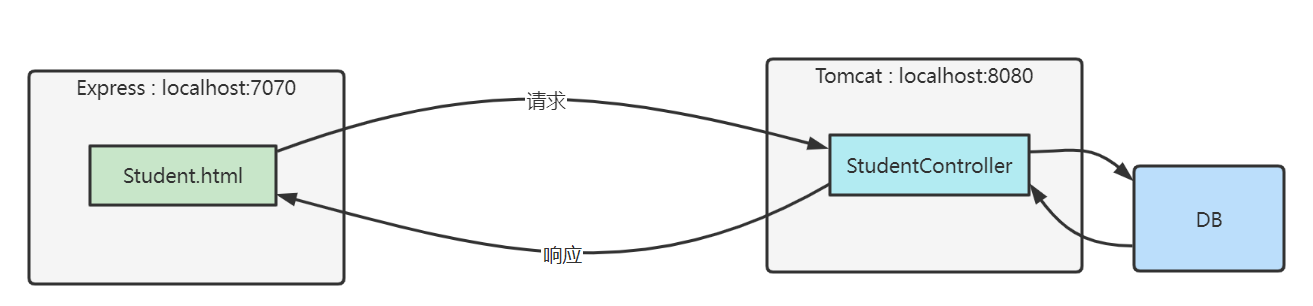• 前端只有静态页面，使用 Express 服务器
• 后端使用 Tomcat 服务器，通过 SpringBoot、MyBatis 等框架获取数据库数据

#### 1) 查找元素

• document.getElementById - 根据 id 值查找一个元素
• [document|元素].querySelector - 根据选择器查找第一个匹配元素
• [document|元素].querySelectorAll - 根据选择器查找所有匹配元素

``````<div>
<div class="title">学生列表</div>
<div class="row bold">
<div class="col">编号</div>
<div class="col">姓名</div>
<div class="col">性别</div>
<div class="col">年龄</div>
</div>
</div>
<div class="tbody">
<div class="row">
<div class="col">1</div>
<div class="col">张三</div>
<div class="col">男</div>
<div class="col">18</div>
</div>
</div>
</div>
``````

``````document.querySelector('.title'); // 找到 <div class="title">学生列表</div>
``````

``````document.querySelector('.col'); // 找到 <div class="col">编号</div>
``````

``````document.querySelectorAll('.col');

/*
找到的是一个集合
<div class="col">编号</div>
<div class="col">姓名</div>
<div class="col">性别</div>
<div class="col">年龄</div>
<div class="col">1</div>
<div class="col">张三</div>
<div class="col">男</div>
<div class="col">18</div>
*/
``````

``````const thead = document.querySelector('.thead');

/*
找到的是一个集合
<div class="col">编号</div>
<div class="col">姓名</div>
<div class="col">性别</div>
<div class="col">年龄</div>
*/
``````

``````document.getElementById("id值")
``````

``````document.querySelector("#id值")
``````

#### 2) 修改元素内容

• 元素.innerHTML
• 元素.textContent

``````document.querySelector('.title').innerHTML = '侠客列表'
``````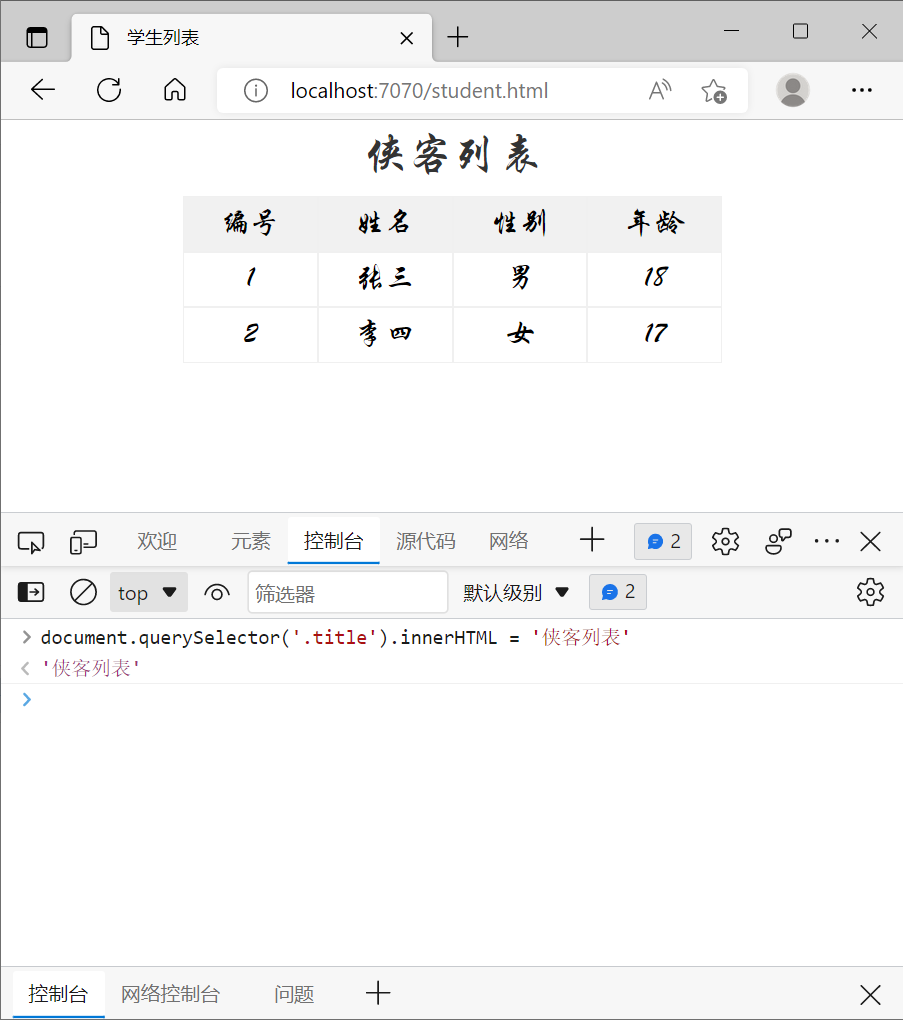innerHTML 会解析内容中的标签，例如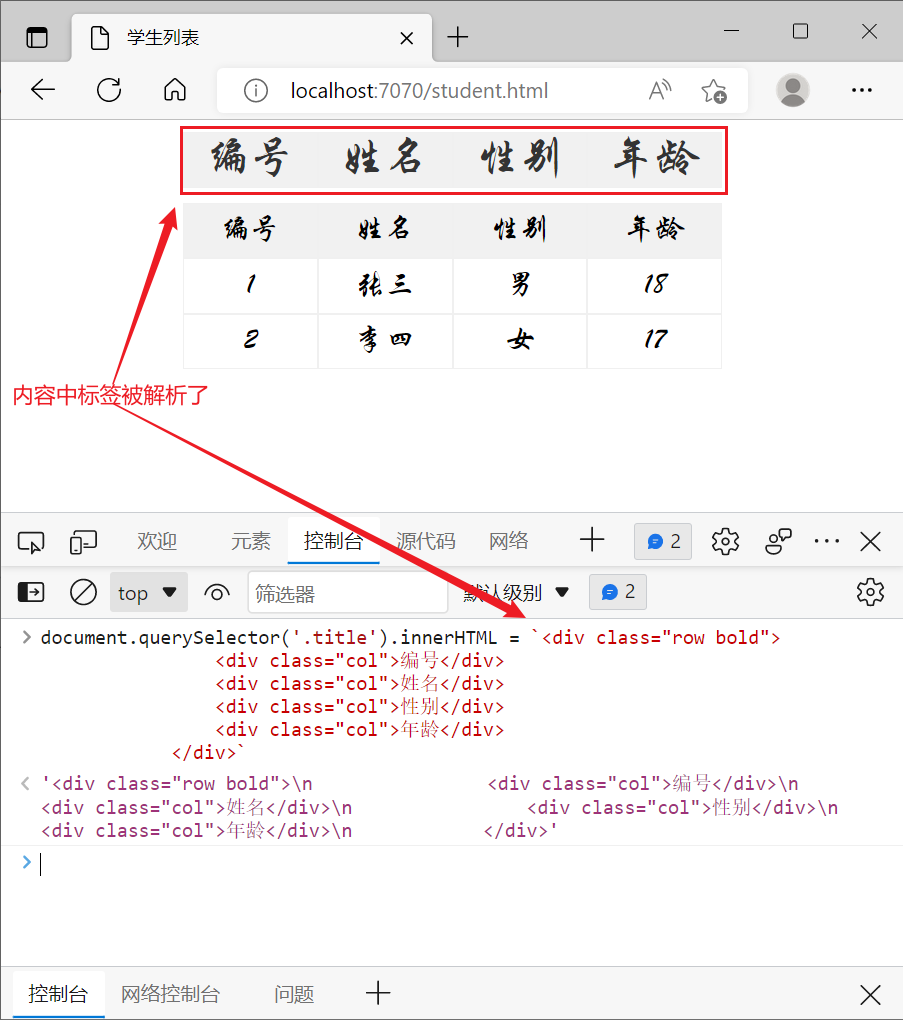textContext 不会解析内容中的标签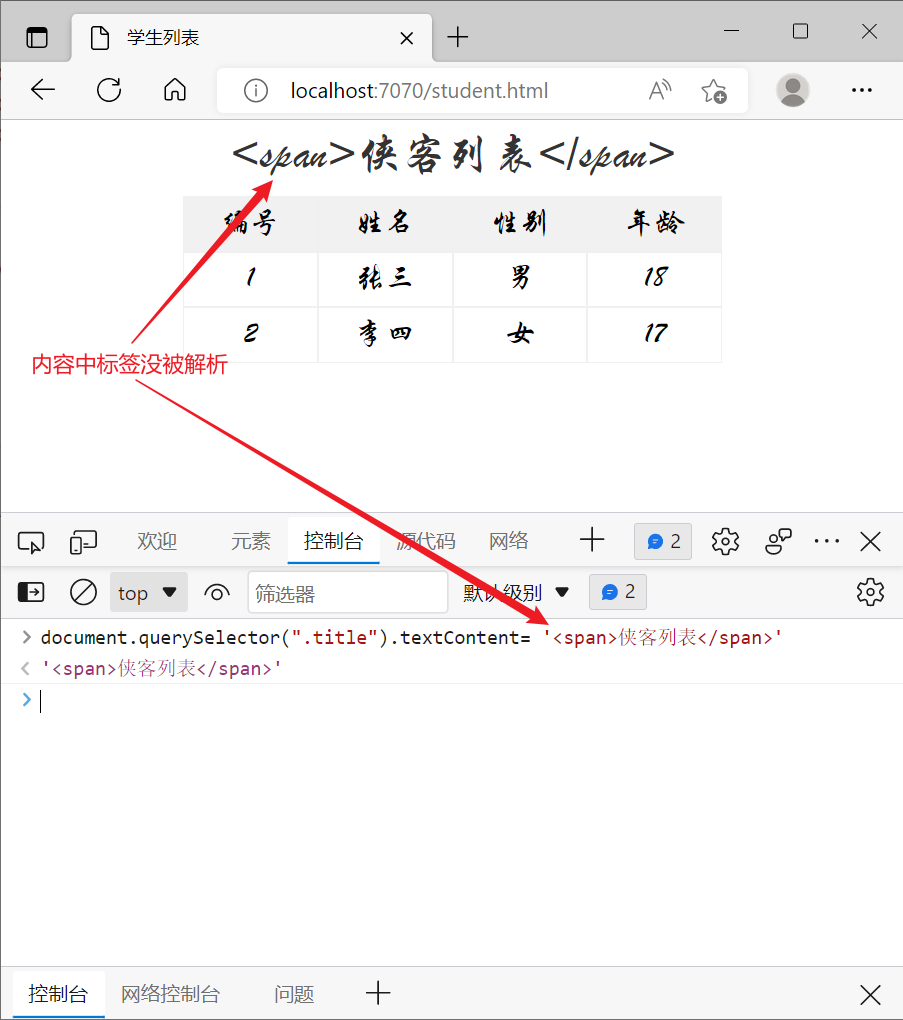#### 3) 利用模板

``````<div>
<div class="title">学生列表</div>
<div class="row bold">
<div class="col">编号</div>
<div class="col">姓名</div>
<div class="col">性别</div>
<div class="col">年龄</div>
</div>
</div>
<div class="tbody">
</div>
</div>

<template id="tp">
<div class="row">
<div class="col">xx</div>
<div class="col">xx</div>
<div class="col">xx</div>
<div class="col">xx</div>
</div>
</template>

<script>
// 将来这些数据从 java 端返回
let array = [
{ id: 1, name: '张三', sex: '男', age: 18 },
{ id: 2, name: '李四', sex: '女', age: 17 }
];

const tp = document.getElementById("tp");
const row = tp.content;
const [c1,c2,c3,c4] = row.querySelectorAll(".col");
const tbody = document.querySelector('.tbody');
for(const {id,name,sex,age} of array) {
c1.textContent = id;
c2.textContent = name;
c3.textContent = sex;
c4.textContent = age;
// 复制元素
const newRow = document.importNode(row, true);
// 建立父子关系，左边父，右边子
tbody.appendChild(newRow);
}
</script>
``````

#### 4) Fetch API

Fetch API 可以用来获取远程数据，它有两种方式接收结果，同步方式与异步方式

``````fetch(url, options) // 返回 Promise
``````

``````const 结果 = await Promise
// 后续代码
``````
• await 关键字必须在一个标记了 async 的 function 内来使用
• 后续代码不会在结果返回前执行

``````Promise
.then(结果 => { ... })
// 后续代码
``````
• 后续代码不必等待结果返回就可以执行

``````[
{ "id": 1, "name": "张三", "sex": "男", "age": 18 },
{ "id": 2, "name": "李四", "sex": "女", "age": 17 }
]
``````

``````<script>
async function findStudents() {
try {
// 获取响应对象
const resp = await fetch('students.json')

// 获取响应体, 按json格式转换为js数组
const array = await resp.json();

// 显示数据
const tp = document.getElementById("tp");
const row = tp.content;
const [c1,c2,c3,c4] = row.querySelectorAll(".col");
const tbody = document.querySelector('.tbody');
for(const {id,name,sex,age} of array) {
c1.textContent = id;
c2.textContent = name;
c3.textContent = sex;
c4.textContent = age;
// 复制元素
const newRow = document.importNode(row, true);
// 建立父子关系
tbody.appendChild(newRow);
}
} catch (e) {
console.log(e);
}

}
findStudents()
</script>
``````
• fetch(‘students.json’) 内部会发送请求，但响应结果不能立刻返回，因此 await 就是等待响应结果返回
• 其中 resp.json() 也不是立刻能返回结果，它返回的也是 Promise 对象，也要配合 await 取结果

``````<script>
fetch('students.json')
.then( resp => resp.json() )
.then( array => {
// 显示数据
const tp = document.getElementById("tp");
const row = tp.content;
const [c1,c2,c3,c4] = row.querySelectorAll(".col");
const tbody = document.querySelector('.tbody');
for(const {id,name,sex,age} of array) {
c1.textContent = id;
c2.textContent = name;
c3.textContent = sex;
c4.textContent = age;
// 复制元素
const newRow = document.importNode(row, true);
// 建立父子关系
tbody.appendChild(newRow);
}
})
.catch( e => console.log(e) )

</script>
``````
• 第一个 then 是在响应返回后，才会调用它里面的箭头函数，箭头函数参数即 resp 响应对象
• 第二个 then 是在 json 解析完成后，才会调用它里面的箭头函数，箭头函数参数即解析结果（本例是 array 数组）
• 上一个 then 返回的是 Promise 对象时，才能链式调用下一个 then
##### 跨域问题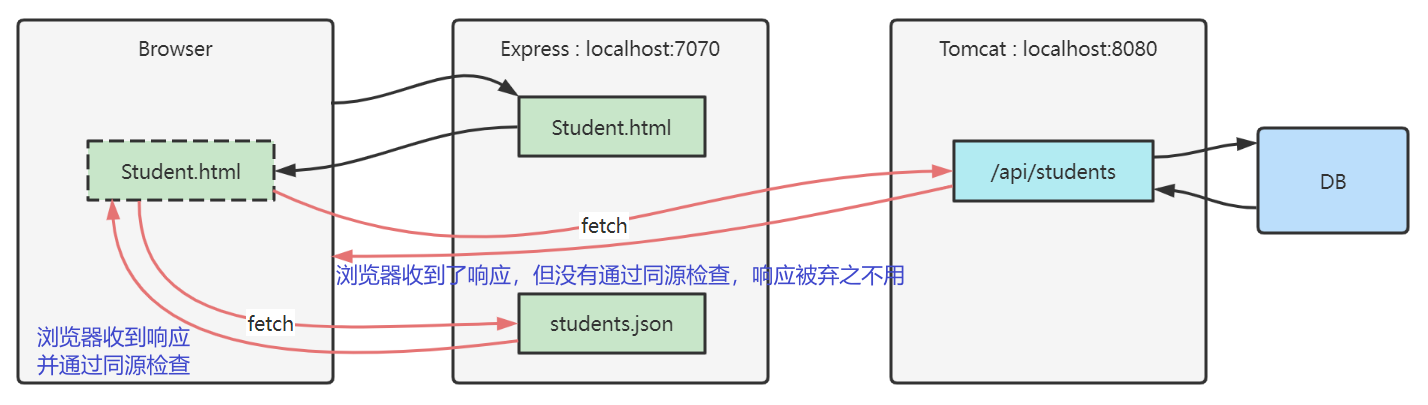• 只要协议、主机、端口之一不同，就不同源，例如
• 同源检查是浏览器的行为，而且只针对 fetch、xhr 请求
• 如果是其它客户端，例如 java http client，postman，它们是不做同源检查的
• 通过表单提交、浏览器直接输入 url 地址这些方式发送的请求，也不会做同源检查
• 更多相关知识请参考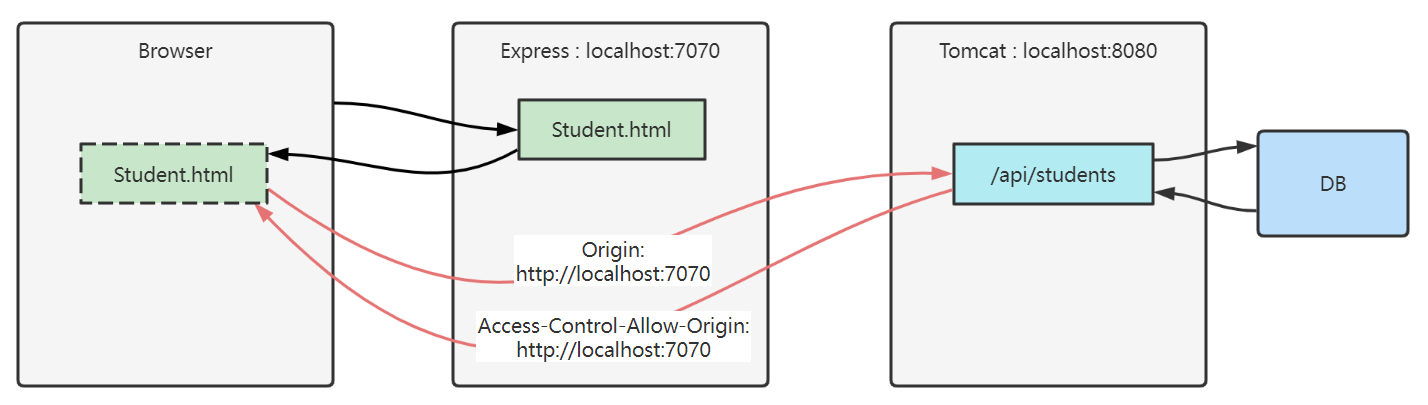• fetch 请求跨域，会携带一个 Origin 头，代表【发请求的资源源自何处】，目标通过它就能辨别是否发生跨域
• 我们的例子中：student.html 发送 fetch 请求，告诉 tomcat，我源自 localhost:7070
• 目标资源通过返回 Access-Control-Allow-Origin 头，告诉浏览器【允许哪些源使用此响应】
• 我们的例子中：tomcat 返回 fetch 响应，告诉浏览器，这个响应允许源自 localhost:7070 的资源使用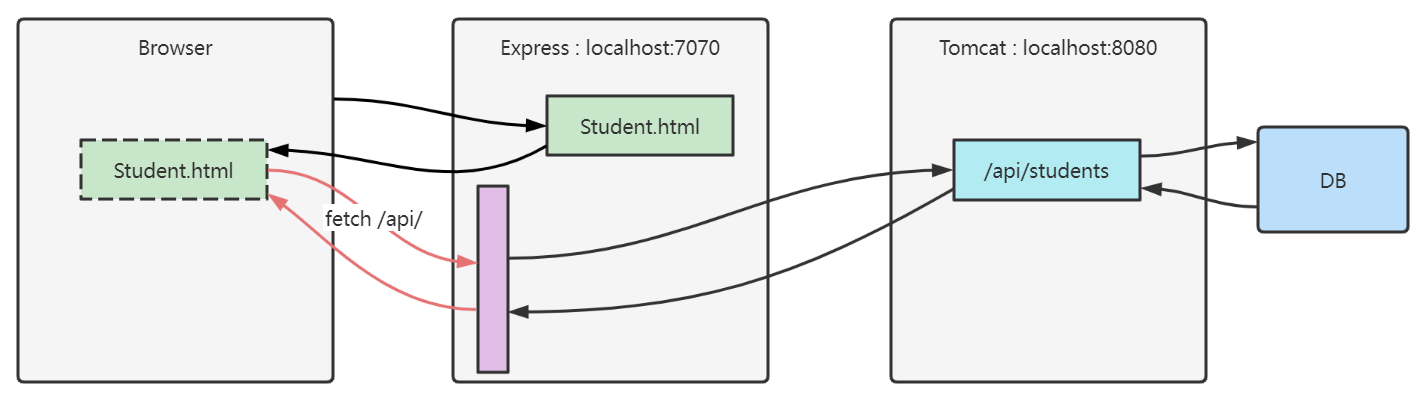``````npm install http-proxy-middleware --save-dev
``````

``````import {createProxyMiddleware} from 'http-proxy-middleware'

// ...

app.use('/api', createProxyMiddleware({ target: 'http://localhost:8080', changeOrigin: true }));
``````

fetch 代码改为

``````const resp = await fetch('http://localhost:7070/api/students')
``````

``````const resp = await fetch('/api/students')
``````

#### 5) 模块化

``````export const a = 10;
export let b = 20;
export function c() {
console.log('c');
}
``````

``````const a = 10;
let b = 20;
function c() {
console.log('c')
}

export {a,b,c}
``````

``````export const a = 10;
export let b = 20;
export function c() {
console.log('c')
}

export default b;
``````

import 语法

``````<script type="module">
import 语句
</script>
``````
• import 需要遵循同源策略

``````import * as module from '/1.js'
console.log(module.a)		// 输出10
console.log(module.b)		// 输出20
module.c()					// 输出c
``````

``````import {a,c} from '/1.js'
console.log(a)				// 输出10
c()							// 输出c
``````

``````import x from '/1.js'
console.log(x)				// 输出20
``````